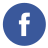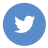### How can you display Typing Speed using Javascript or the jQuery library

javascript

To display the typing speed using JavaScript or the jQuery library, you can use a keydown event handler to track the keys that the user is pressing and a timer to calculate the elapsed time.

Here is an example of how you can do this using JavaScript:

``````<!-- create an input element to track the user's typing -->
<input id="typing-input" type="text" />

<!-- create a paragraph element to display the typing speed -->
<p id="typing-speed"></p>

<script>
// create a variable to track the number of keystrokes
let keystrokes = 0;

// create a variable to track the start time
let startTime;

// create a variable to track the elapsed time
let elapsedTime = 0;

// create a variable to track the typing speed
let typingSpeed = 0;

// get a reference to the input element and the typing speed element
const input = document.getElementById('typing-input');
const speedElement = document.getElementById('typing-speed');

// add a keydown event listener to the input element
// increment the keystroke count
keystrokes++;

// if this is the first keystroke, record the start time
if (keystrokes === 1) {
startTime = new Date().getTime();
}
});

// add an input event listener to the input element
// calculate the elapsed time
elapsedTime = new Date().getTime() - startTime;

// calculate the typing speed
typingSpeed = Math.round(keystrokes / (elapsedTime / 1000));

// update the typing speed element
speedElement.textContent = `Typing speed: \${typingSpeed} wpm`;
});
</script>``````

This example uses the keydown event to track the keys that the user is pressing and the input event to calculate the elapsed time and update the typing speed display.